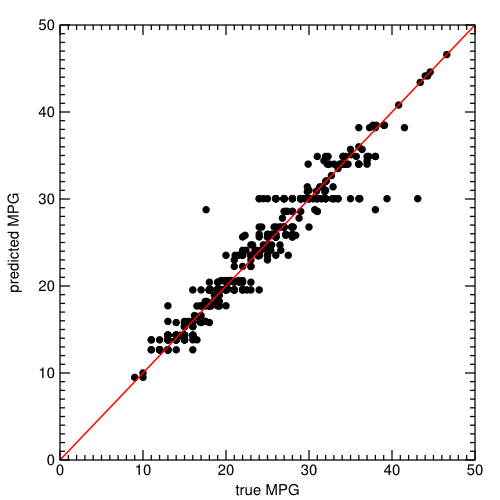Home > Hadrian > Before you begin…

## Before you begin…

Download and install Titus and Numpy. This article was tested with Titus 0.8.2 and Numpy 1.8.2; newer versions should work with no modification. Python >= 2.6 and < 3.0 is required.

Launch a Python prompt and import `csv` and `titus.producer.cart`:

``````Python 2.7.6
>>> import csv
>>> import titus.producer.cart as cart
``````

Download the MPG dataset. This is a tab-separated values file in which the first line contains field names.

Learn about categorical and regression trees (CART) if you’re not already familiar with it.

Tree learning is applicable to many different types of data— continuous and categorical predictors, continuous and categorical predictands, even structured predictands like vectors (beyond the scope of this tutorial, but not beyond the scope of PFA). Titus has a `cart.Dataset` class to codify this structure, and our first task will be to put the input data in this structure.

Start by defining the field names, field order, and field types for the scoring engine’s input. The scoring engine does not include the “mpg” field (because it’s supposed to predict that!) or the “carType” (which is unique for each data entry). We’ll express this list of fields using Avro, so that we don’t have to redefine it later.

``````# input for the scoring engine
inputType = {"type": "record",
"name": "Input",
"fields": [{"name": "cylinders",    "type": "string"},
{"name": "displacement", "type": "double"},
{"name": "horsepower",   "type": "double"},
{"name": "weight",       "type": "double"},
{"name": "acceleration", "type": "double"},
{"name": "modelYear",    "type": "double"},
{"name": "origin",       "type": "string"}]}

fieldNamesInFile = ["mpg"] + [x["name"] for x in inputType["fields"]] + ["carName"]
fieldNamesForTree = fieldNamesInFile[:-1]
fieldTypesForTree = [float] + [float if x["type"] == "double" else str for x in inputType["fields"]]

def datasetGenerator(fileName):
assert file.next() == fieldNamesInFile
# drop the last column ("car name") and assign data types to each row
for row in file:
yield [t(x) for t, x in zip(fieldTypesForTree, row[:-1])]

dataset = cart.Dataset.fromIterable(datasetGenerator("auto-mpg.tsv"),
names=fieldNamesForTree)
``````

Titus loads the data into Numpy arrays, using an integer array and index-based lookup for the categorical strings. (If a string value appears multiple times, its content is stored only once, which is good for large datasets with categories that have only a few unique values.)

## Decision tree learning

Now we declare the tree using this dataset (`fromWholeDataset` means that the predictand, “mpg”, is one of the fields in `dataset` and should not be used for prediction; the unnamed constructor assumes the dataset contains predictors only and takes the predictand from another source).

``````decisionTree = cart.TreeNode.fromWholeDataset(dataset, predictandName="mpg")
``````

The tree has been declared, but it currently contains only one node, a single leaf pointing to all of the data. To perform tree-based learning, we must recursively split the data in such a way as to maximize a gain term.

The default gain terms are given by the following methods of the `cart.TreeNode` class, which can be overridden by a subclass.

• string-valued predictand (decision tree), string-valued regressor: `categoricalEntropyGainTerm`
• string-valued predictand (decision tree), numerical regressor: `numericalEntropyGainTerm`
• numerical predictand (regression tree), string-valued regressor: `categoricalNVarianceGainTerm`
• numerical predictand (regression tree), numerical regressor: `numericalNVarianceGainTerm`

The stopping condition, which tells Titus when to stop splitting tree nodes, is given by a user-supplied function of `node` and `depth`.

``````def stoppingCondition(node, depth):
# optionally print diagnostic information while splitting
print node.datasetSize, depth, node.nTimesVariance, node.gain
if node.nTimesVariance < 100 or depth > 10:
return False  # stop splitting
else:
return True   # continue splitting

# now perform the recursive splitting
decisionTree.splitUntil(stoppingCondition)
``````

## PFA output

The tree is complete. You can now create PFA from it, either using `pfaDocument` to get a complete scoring engine or `pfaValue` to get a partial document, for use with customized preprocessing.

``````pfaDocument = decisionTree.pfaDocument(inputType, "TreeNode")

import titus.producer.tools as t

import json
``````

## Testing and tree-diagnostics

To do a quick test, we can create a scoring engine in Titus and run the predictor.

``````from titus.genpy import PFAEngine

# read the training data back in to verify that the model reproduces it
file.next()   # get past the field names
for row in file:
datum = {n: t(x) for n, t, x in zip(fieldNamesForTree, fieldTypesForTree, row[:-1])}
predictedMPG = engine.action(datum)
trueMPG = datum["mpg"]
print predictedMPG, trueMPG  # could plot these points
``````The fact that the model reproduces the training data is not sufficient for a real data analysis (cross-validate!), but it’s enough for this simple example. To get a sense of whether we’ve overtrained, compute some basic features of the tree itself (number of leaves « training set size is a good start).

``````# what's the tree's complexity (number of leaves, depth, etc)?
numberOfLeaves = len(list(decisionTree.walkLeaves()))
minDepth = min(depth for node, depth in decisionTree.walkLeaves())
maxDepth = max(depth for node, depth in decisionTree.walkLeaves())
print numberOfLeaves, minDepth, maxDepth

# which fields are split on, and how often?
from collections import Counter
counter = Counter()
for node, depth in decisionTree.walkNodes():
if not splitField(node) is None:
counter[splitField(node)] += 1
print counter
``````